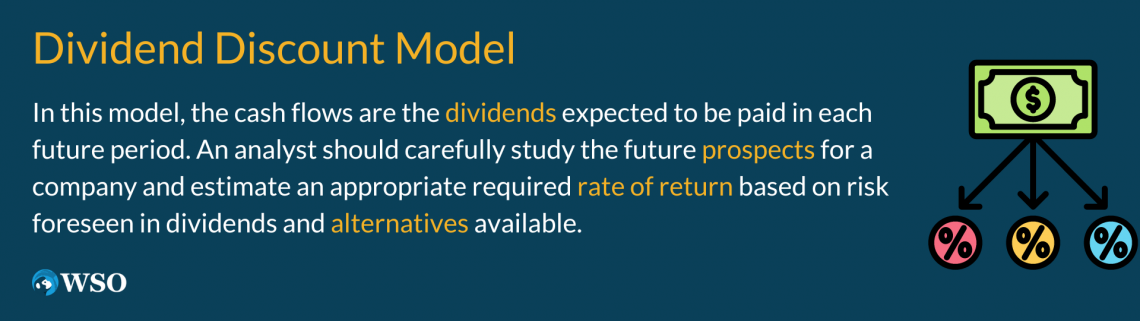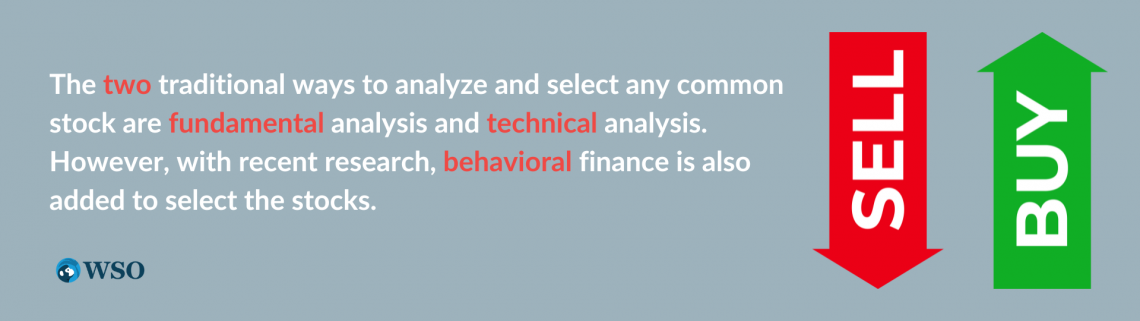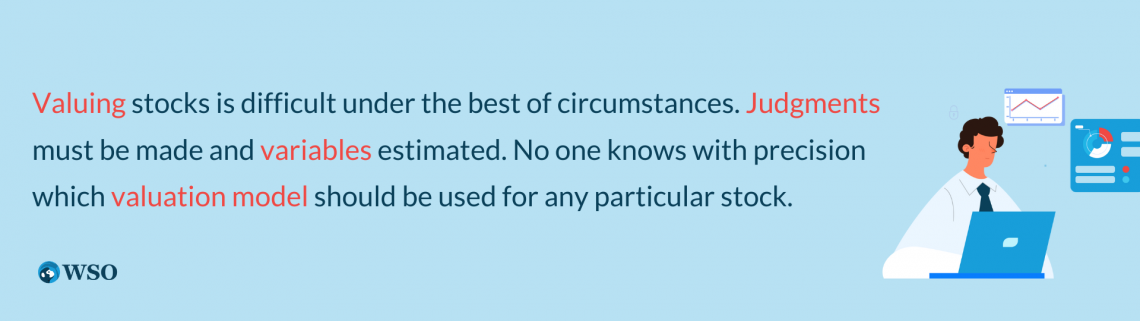# Common Stock

It represents the ownership interest of corporations. Closely held are those firms' shares that only a few individuals hold. Go public are those companies that sell common stock to the general public.

It represents the ownership interest of corporations. Closely held are those firms' shares that only a few individuals hold. Go public are those companies that sell common stock to the general public.It depends primarily on the additional capital needed by the firm. If a corporation meets certain requirements, it may choose to be listed on one or more exchanges or, otherwise, will be listed in the over-the-counter exchange.

As an owner of the common stock, they are entitled to elect the corporation's directors and vote on major issues. Each owner can cast votes equal to the number of shares they own.

Stockholders have limited liability, meaning they cannot lose more than their investment in their corporation.

Dividends are the only cash payment made to the stockholders regularly. The following dividend terms are important:

• The Dividend Yield: the income component of a stock's return stated on a percentage basis.
• The Payout Ratio: the ratio of dividends to earnings. It indicates the percentage of a firm's earnings paid out in cash to its stockholders.

## Note

The basic approaches to valuing common stocks using fundamental analysis are Discounted cash flow techniques, including the dividend discount model and relative valuation.

## Discounted Cash Flow Techniques, Common Stock & Financial Securities

The security value can be estimated by a present value process involving the capitalization (discounting) of expected future cash flows, known as the capitalization of income method.Where

• k = appropriate discount rate or required rate of return
• t = time of future cash flows

Just as the value of a bond is the present value of any interest payments plus the present value of the bond's face value that will be received at maturity, the value of the common stock is the present value of all the cash flows to be received from the issuer (corporation).

The questions that arise are:

•  What cash flows are used to value a stock?
•  What are the expected amounts of the cash flows?
•  When will the expected cash flows be received?

For example, an investor with an initial investment of 100000 receives a different amount of expected benefits (cash inflows) as follows:

Calculation Of Discounted Cash Flows
ParticularsYear 0Year 1Year 3Year 4Year 5
Cash flows-10000015000250003500035000
Discount factor @ 6%10.9430.8900.8400.792
Discounted cash flow-10000014145222502940027720

Discounted cash flow (net) = -100000 + 14145 + 22250 + 29400 + 27720 = -6485

Thus, the net present value is negative and should not go ahead.

## Dividend discount model

In this model, the cash flows are the dividends expected to be paid in each future period. An analyst should carefully study the prospects for a company and estimate an appropriate required rate of return based on risk foreseen in dividends and alternatives available.Where

• D1, D2 …= the dividends expected to be received in each future period.
• k = the required rate of return

Two immediate problems with this equation:

• The infinity assumption

The last term in the equation indicates that investors are dealing with infinity. Since the common stock has no maturity date, they must value a stream of dividends that may be paid forever.

• The dividend stream is uncertain.

No specified number of dividends, if any, are paid. Dividends must be declared periodically by the firm's board of directors. The dividends for most firms are expected to grow over time.

The first problem will be solved when we deal with the second problem, specifying the expected stream of dividends. The conventional solution for the number of dividends expected to grow over time is to make assumptions about the expected growth rate.The three growth rate models for dividends are

1. The zero growth rate case

A dividend stream with a zero growth rate resulting from a fixed dividend equal to the current dividend paid every year from now to infinity.

Estimated value of stock = D0 / k

Where D0 is the constant dividend expected, and k is the required rate of return for this particular common stock.

2. The constant growth rate case

A dividend stream that is growing at a constant rate g, starting with

Estimated value of stock = Estimated value of stock = D1 / (k-g)

D1 is the dividend expected to be received at the end of year one, and g is the expected growth.

## Note

k must be greater than g; otherwise, nonsense results are produced.

3. The multiple growth rate case

A dividend stream that is growing at variable rates. Estimated value = PV of the dividends during the period of unusual growth + PV of the terminal price (which is a function of all constant growth)

Pn = Dn+1 / (k-g)

Because Pn is the estimated price of the stock at the end of period n, it must be discounted back to the present. When it is added to the value of discounted dividends from the first term, we have the estimated value of the stock today.

## Relative valuation techniques

An alternative fundamental analysis approach often used by practicing security analysts is relative valuation techniques.The relative value concept is based on making comparisons to determine value by calculating measures such as the P/E ratio, price to book, price to sales, and economic value added.

1. P/E ratio (earning multiplier approach)

The number of times investors value earnings as expressed in the stock prices.

For example, a stock priced at \$100, with the most recent 12-month earnings of \$5, is said to be selling for a multiple of 20. In contrast, if another stock had \$2.50 and sold for \$100, investors would value the stock at 40 times earnings.

P0 = current stock price = E0 × P0 / E0

P0 is the current stock price, and E0 is the most recent 12-month EPS.

P/E ratios reflect investors' expectations about the growth potential of a stock and the risk involved. Other things being equal, the greater the risk of a stock, the lower the P/E ratio; however, growth prospects may offset the risk and lead to a higher P/E ratio.

It reflects investor optimism and pessimism. It is inversely related to the required rate of return. The required rate of return, in turn, is positively related to the interest rates.

2. Price to book value

It is calculated as the price to stockholders' equity ratio as measured on the balance sheet. Investors must be careful when interpreting this ratio.

For example, firms with significant "intellectual property" may have a low book value. On the other hand, banks tend to have easily valued investments close to book value, so their ratios are close to 1.0.

## Note

Other issues to consider are share repurchases by a company and restructurings, which tend to raise the price-to-book ratio.

3. Price/Sales ratio (PSR)

It is calculated by dividing a company's current stock price by its revenue per share over the four more recent quarters. It indicates what the market will pay for a firm's revenue.

Investors may be concerned about the P/E ratio because a company could have erratic earnings. In contrast, the PSR ratio can be used to value any public company and companies with no earnings.

Furthermore, many believe sales are unlikely to be "managed" by the company than earnings.

An often-quoted rule of thumb is that a PSR of 1.0 is average for all companies, and therefore those with a PSR considerably less than 1.0, such as 0.5, are bargains. It is important to interpret the ratio within industry bounds.

For example, retailers tend to have low price/sales ratios because of their low margins, whereas biotechnology companies tend to have high price/sales ratios.

It is the difference between operating profits and a company's true cost of capital for debt and equity and reflects an emphasis on return on capital. If this difference is positive, the company has added value.

Investors interested in this approach are to search for companies with a return on capital over 20 percent, as this will all likely exceed the cost of capital and add value to the company.

## Approaches for analyzing and selecting stocks

Fundamental and technical analysis are the two traditional ways to analyze and select any common stock. However, with recent research, behavioral finance is also added to select the stocks.1. Technical analysis

It is the methodology of forecasting fluctuations in securities prices applied to individual securities or the market as a whole (Dow Jones industrial average). It is based on the rationale that the functions of demand and supply determine the value of a stock.

Price and volume are primary tools of the technical analyst, as are various technical indicators.

## Note

Technical analysis involves using charts of price patterns to detect trends believed to persist over time.

2. Fundamental analysis

It is based on the premise that any security has an intrinsic value estimated by the investors. This value is a function of the firm's underlying financial statements and various variables that combine to predict the expected rate of return.

The price prevailing in the market is called "market price (MP)," and the one justified by its fundamentals is called "intrinsic value (IV)" of trading rules/recommendations.

• if IV > MP, buy the security;
• if IV < MP, sell the security; and
• if IV = MP, no action (remain neutral)

3. Behavioral finance implications

Traditional economics is built on the proposition that investors act rationally based on utility theory. Behavioral finance recognizes that investors can behave irrationally.

Markets overreact, both up and down. Investors are motivated by numerous "irrational" forces, such as overconfidence, regrets about decisions, aversion to losses, etc.

## Note

This means careful attention to past trends and similar information can be beneficial.

## Summary

Valuing stocks is difficult under the best circumstances. Judgments must be made and variables estimated. No one knows which valuation model should be used for any particular stock.It is almost impossible to prove that an investor's calculations for a valuation model are correct or incorrect (although many calculations could be judged by most people to be "reasonable" or not "reasonable").

Valuation of stocks will continue to be an art and not a science. Errors are to be expected. In the final analysis, stocks are worth what investors pay for them. Valuations may appear out of line, but market prices prevail.

Fundamental and technical analysis are two primary approaches for analyzing and selecting common stocks. Efficient market considerations should be considered.

The relative value concept is based on making comparisons to determine value.

By calculating measures such as the P/E ratio and comparing them to some benchmark(s), analysts can avoid having to estimate the g and k parameters of the Dividend discount model and one-point estimates of the value of a stock in the form of intrinsic values.

The present value approach for common stocks is similar to that used with bonds. A required expected rate of return must be determined based on the risk-free rate and a risk premium.### Everything You Need To Master Excel Modeling

To Help You Thrive in the Most Prestigious Jobs on Wall Street.

Researched and authored by Riya Choudhary | Linkedin

Reviewed and edited by Parul Gupta | LinkedIn